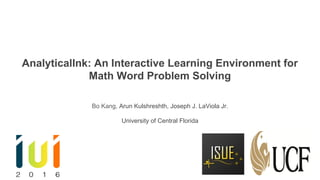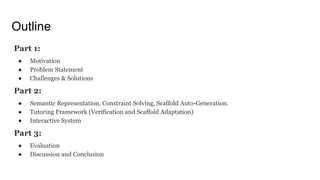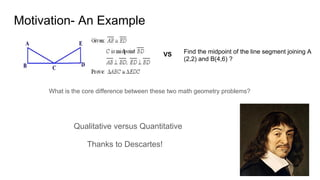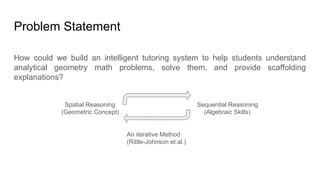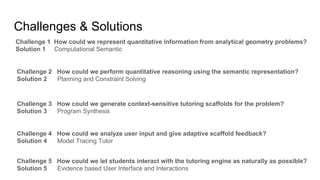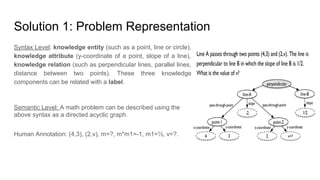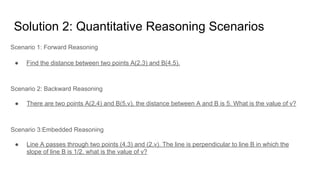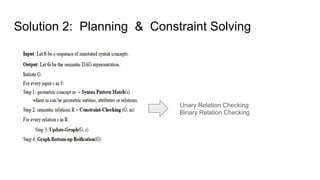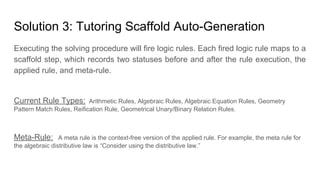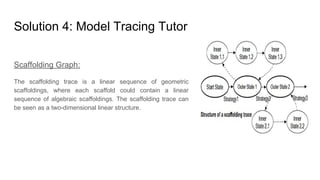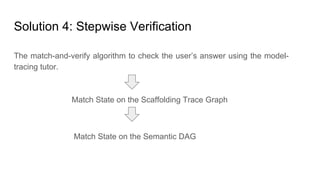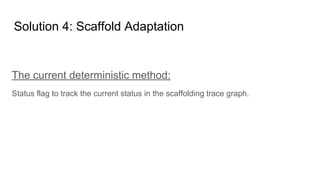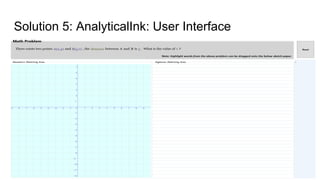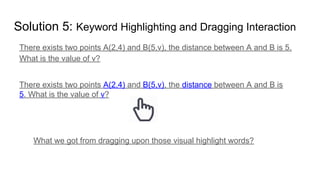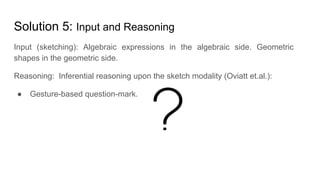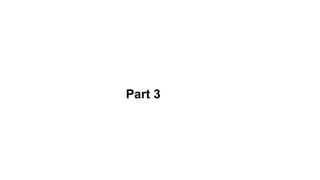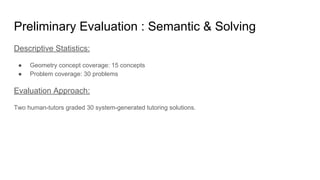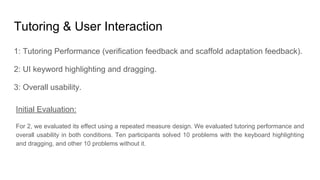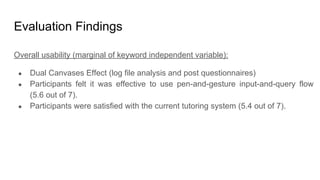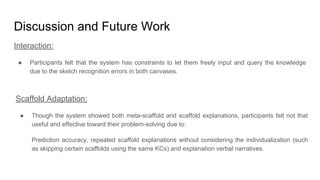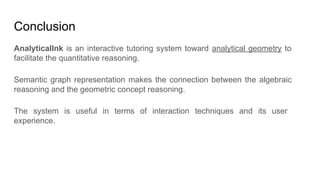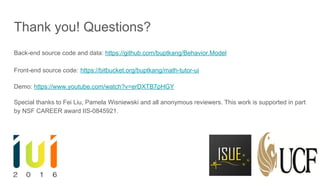1 of 25

### IUI 2016 Presentation Slide

1. AnalyticalInk: An Interactive Learning Environment for Math Word Problem Solving Bo Kang, Arun Kulshreshth, Joseph J. LaViola Jr. University of Central Florida
2. Outline Part 1: ● Motivation ● Problem Statement ● Challenges & Solutions Part 2: ● Semantic Representation, Constraint Solving, Scaffold Auto-Generation. ● Tutoring Framework (Verification and Scaffold Adaptation) ● Interactive System Part 3: ● Evaluation ● Discussion and Conclusion
3. Part 1
4. Motivation- An Example What is the core difference between these two math geometry problems? Find the midpoint of the line segment joining A (2,2) and B(4,6) ? VS Qualitative versus Quantitative Thanks to Descartes!
5. Problem Statement How could we build an intelligent tutoring system to help students understand analytical geometry math problems, solve them, and provide scaffolding explanations? Spatial Reasoning (Geometric Concept) Sequential Reasoning (Algebraic Skills) An iterative Method (Rittle-Johnson et.al.)
6. Challenges & Solutions Challenge 1 How could we represent quantitative information from analytical geometry problems? Solution 1 Computational Semantic Challenge 2 How could we perform quantitative reasoning using the semantic representation? Solution 2 Planning and Constraint Solving Challenge 3 How could we generate context-sensitive tutoring scaffolds for the problem? Solution 3 Program Synthesis Challenge 4 How could we analyze user input and give adaptive scaffold feedback? Solution 4 Model Tracing Tutor Challenge 5 How could we let students interact with the tutoring engine as naturally as possible? Solution 5 Evidence based User Interface and Interactions
7. Part 2
8. Solution 1: Problem Representation Syntax Level: knowledge entity (such as a point, line or circle), knowledge attribute (y-coordinate of a point, slope of a line), knowledge relation (such as perpendicular lines, parallel lines, distance between two points). These three knowledge components can be related with a label. Semantic Level: A math problem can be described using the above syntax as a directed acyclic graph. Human Annotation: (4,3), (2,v), m=?, m*m1=-1, m1=½, v=?.
9. Solution 2: Quantitative Reasoning Scenarios Scenario 1: Forward Reasoning ● Find the distance between two points A(2,3) and B(4,5). Scenario 2: Backward Reasoning ● There are two points A(2,4) and B(5,v), the distance between A and B is 5. What is the value of v? Scenario 3:Embedded Reasoning ● Line A passes through two points (4,3) and (2,v). The line is perpendicular to line B in which the slope of line B is 1/2. what is the value of v?
10. Solution 2: Planning & Constraint Solving Unary Relation Checking Binary Relation Checking
11. Solution 3: Tutoring Scaffold Auto-Generation Executing the solving procedure will fire logic rules. Each fired logic rule maps to a scaffold step, which records two statuses before and after the rule execution, the applied rule, and meta-rule. Current Rule Types: Arithmetic Rules, Algebraic Rules, Algebraic Equation Rules, Geometry Pattern Match Rules, Reification Rule, Geometrical Unary/Binary Relation Rules. Meta-Rule: A meta rule is the context-free version of the applied rule. For example, the meta rule for the algebraic distributive law is “Consider using the distributive law.”
12. Solution 4: Model Tracing Tutor Scaffolding Graph: The scaffolding trace is a linear sequence of geometric scaffoldings, where each scaffold could contain a linear sequence of algebraic scaffoldings. The scaffolding trace can be seen as a two-dimensional linear structure.
13. Solution 4: Stepwise Verification The match-and-verify algorithm to check the user’s answer using the model- tracing tutor. Match State on the Scaffolding Trace Graph Match State on the Semantic DAG
14. Solution 4: Scaffold Adaptation The current deterministic method: Status flag to track the current status in the scaffolding trace graph.
15. Solution 5: AnalyticalInk: User Interface
16. Solution 5: Keyword Highlighting and Dragging Interaction There exists two points A(2,4) and B(5,v), the distance between A and B is 5. What is the value of v? There exists two points A(2,4) and B(5,v), the distance between A and B is 5. What is the value of v? What we got from dragging upon those visual highlight words?
17. Solution 5: Input and Reasoning Reasoning: Inferential reasoning upon the sketch modality (Oviatt et.al.): ● Gesture-based question-mark. Input (sketching): Algebraic expressions in the algebraic side. Geometric shapes in the geometric side.
18. Part 3
19. Preliminary Evaluation : Semantic & Solving Descriptive Statistics: ● Geometry concept coverage: 15 concepts ● Problem coverage: 30 problems Evaluation Approach: Two human-tutors graded 30 system-generated tutoring solutions.
20. Tutoring & User Interaction 1: Tutoring Performance (verification feedback and scaffold adaptation feedback). 2: UI keyword highlighting and dragging. 3: Overall usability. Initial Evaluation: For 2, we evaluated its effect using a repeated measure design. We evaluated tutoring performance and overall usability in both conditions. Ten participants solved 10 problems with the keyboard highlighting and dragging, and other 10 problems without it.
21. Evaluation Findings Tutoring (marginal of keyword highlighting and dragging independent variable): ● Participants used the system to check their answer step. ● Participants used the system to ask the next step. ● Participants made the error-rectification once during the study session. UI keyword highlighting and dragging: ● Participants made less query for the next step using this technique. ● Participants made less error-rectification using this technique. ● Participants preferred to use with the keyword highlighting and dragging UI.
22. Evaluation Findings Overall usability (marginal of keyword independent variable): ● Dual Canvases Effect (log file analysis and post questionnaires) ● Participants felt it was effective to use pen-and-gesture input-and-query flow (5.6 out of 7). ● Participants were satisfied with the current tutoring system (5.4 out of 7).
23. Discussion and Future Work Interaction: ● Participants felt that the system has constraints to let them freely input and query the knowledge due to the sketch recognition errors in both canvases. Scaffold Adaptation: ● Though the system showed both meta-scaffold and scaffold explanations, participants felt not that useful and effective toward their problem-solving due to: Prediction accuracy, repeated scaffold explanations without considering the individualization (such as skipping certain scaffolds using the same KCs) and explanation verbal narratives.
24. Conclusion AnalyticalInk is an interactive tutoring system toward analytical geometry to facilitate the quantitative reasoning. Semantic graph representation makes the connection between the algebraic reasoning and the geometric concept reasoning. The system is useful in terms of interaction techniques and its user experience.
25. Thank you! Questions? Back-end source code and data: https://github.com/buptkang/Behavior.Model Front-end source code: https://bitbucket.org/buptkang/math-tutor-ui Demo: https://www.youtube.com/watch?v=erDXTB7pHGY Special thanks to Fei Liu, Pamela Wisniewski and all anonymous reviewers. This work is supported in part by NSF CAREER award IIS-0845921.